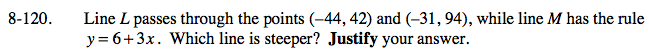### Home > CAAC > Chapter 8 > Lesson 8.3.3 > Problem8-120

8-120.

Line L passes through the points (−44, 42) and (−31, 94), while line M has the rule y = 6 + 3x. Which line is steeper? Justify your answer. Homework Help ✎Since m in y = mx + b is the slope, we know that the slope of line M is 3.
Calculate the slope of line L by either using a slope triangle or by finding the change in y and comparing it to the change in x.

$\frac{42-94}{-44-(-31)}=m$